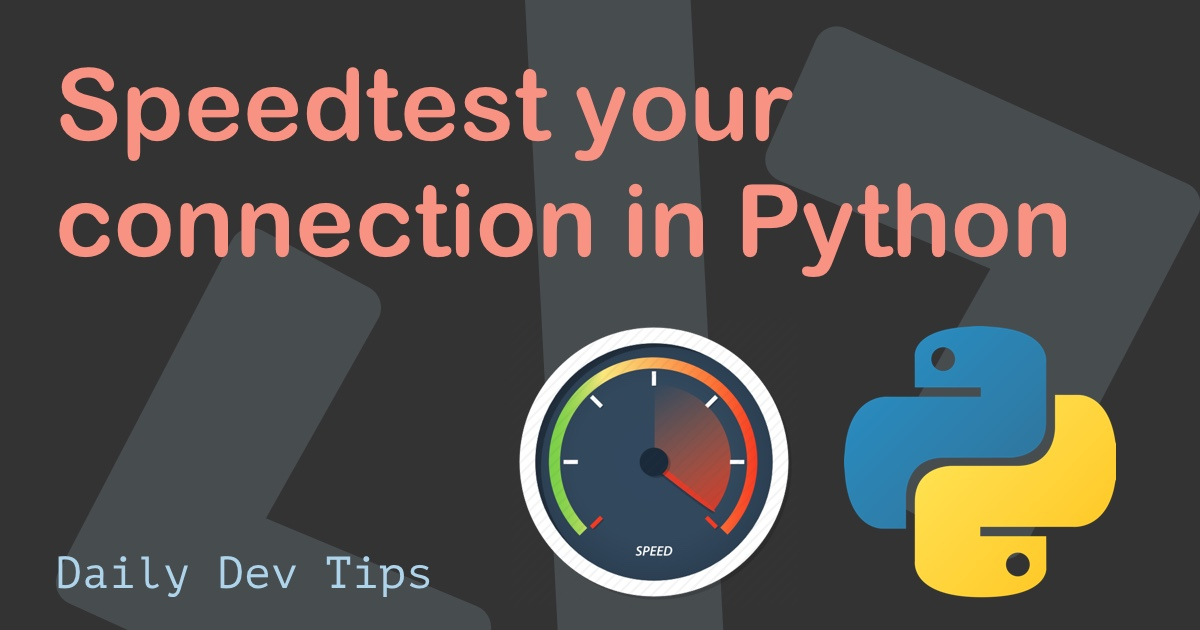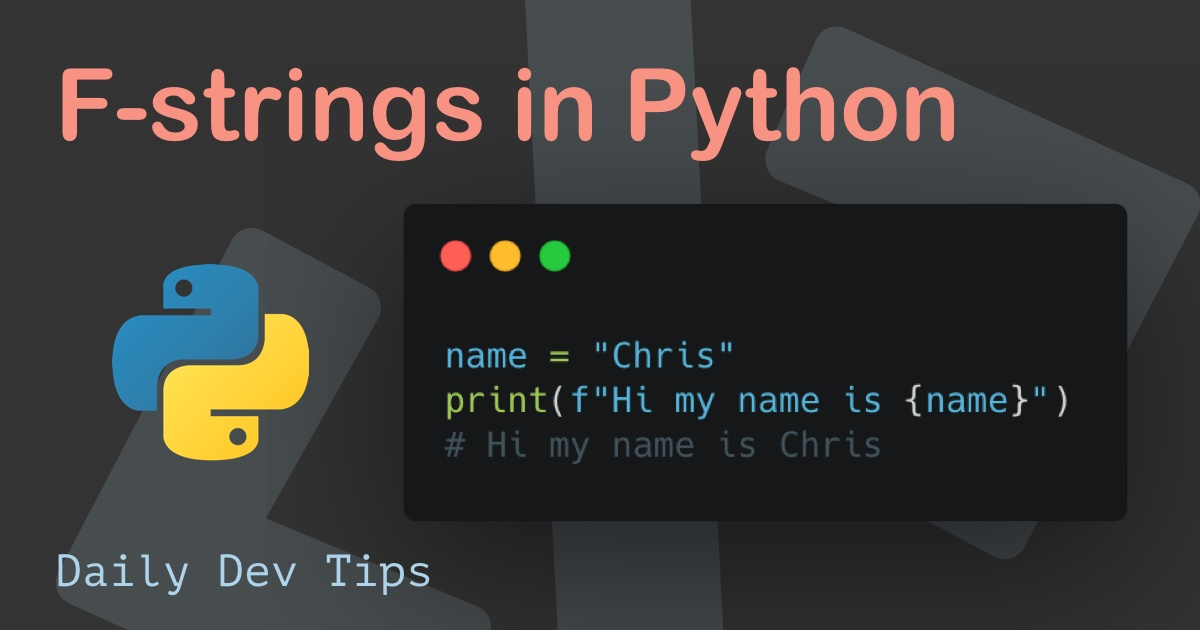Subscribe

# Data types in Python

••••••• + 9 devs liked it

✍️

Looking at the data types in Python

21 May, 2021 · 3 min read

Data types are an essential aspect of a programming language. As it comes down to Python, there are several categorized data types built in that we can leverage.

This article will guide you through the categories and which type each category has.

This guide will show you the basics of each. I will do a more detailed guide on some of these.

## Text type data type in Python permalink

Regarding strings in Python, there is only one option: the `str`.

As single or double quotes define string as we can see here:

``````foo = "String"
bar = 'string'``````

Both variables will hold the exact same string.

## Numeric type data type in Python permalink

With numeric types, there is three built-in option we can leverage.

`int`, `float`, `complex`

I'll first show you what they look like:

``````a = 1 # Int
b = 3.14 # Float
c = 1j # Complex``````

Integers can be numbers of any length and even be negative. The only thing is they can't have decimals.

Some examples of different int in Python.

``````a = 1
b = 83458903489734890
c = -2323434``````

As for a float, this is a number, but it can hold decimals.

``````a = 3.14
b = 1.0
c = -40.53``````

The complex, to me, is a new addition to programming, and it can define an imaginary part determined by the letter `j`.

``````a = 1+2j
b = 5j
c = -3j``````

## Sequence type data type in Python permalink

Sequence types are sets of data. See them as arrays or objects.

There are four basic types we can use.

`list`, `tuple`, `set`, `range`

I'll show you the basics of each sequence.

The list can be used to store multiple items in a single variable. These are generally compared to arrays.

``list = ["dog", "cat", "penguin"]``

The list can store data, change, add or remove.

Tuples, however, can't be changed. This is the main difference from lists. Tuples are also created by using regular brackets.

``tuple = ("dog", "cat", "penguin")``

A set is unordered and unindexed, meaning it can't contain multiple of the same entry. Curly brackets define a set.

``set = {"dog", "cat", "penguin"}``

Then there is also the range option which allows us to create a range of numbers.

``a = range(6)``

This will give us a range from 0-6.

## Mapping type data type in Python permalink

There is another sequence type, but it falls under a mapping type, and it's the `dict` one.

Dictionaries are used to store data as a key-value pair.

``````Dict = {
"type": "pet",
"animal": "dog",
"name": "Yaatree"
}``````

A dictionary is changeable so that items can be removed, added, or changed. We can, however, not have duplicates in a `dict`.

## Boolean type data type in Python permalink

As for the boolean, we know a true/false statement can be used as the `bool` variable.

For instance, we can check random values for True or False.

``````bool(False) # False
bool("a") # True
bool({}) # False
bool(123) # True
bool(0) # False
bool(10 > 9) # True``````

## Data types in Python permalink

You need to know these basic built-in data types of Python when getting started with Python.

I would strongly suggest creating some basic Python script to run these and have a play around with.

Tweet this tip
••••••• + 9 devs liked it

### Speedtest your connection in Python

21 Jun, 2021 · 2 min read### F-strings in Python

7 Jun, 2021 · 2 min readJoin 2086 devs and subscribe to my newsletter

• 1000 articles written
• 2086 devs subscribed
• 529116 words written